# "Additional Loss Due to SWR" (in quotes)

Discussion in 'Antennas, Feedlines, Towers & Rotors' started by AC6LA, Mar 31, 2015.

Not open for further replies.1. From time to time questions arise about using an online calculator to determine the total loss in a length of transmission line. I thought I'd put together a few notes on the subject. Obviously I got a bit carried away.

Most of the online calculators use the ARRL's "Additional Loss Due to SWR" formula, shown (with minor edits) below.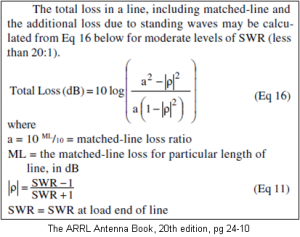The advantage of using this formula is that it is simple and thus easy to implement using JavaScript. That's why so many online calculators all look the same; they all use the same script code in the html of the web page. And they all copied that script from the original (or from each other).

Here's an extract of what the original online calculator looked like, slightly rearranged to save space.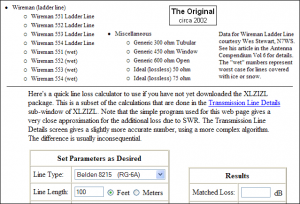And here's an extract from one of many copies currently available, again slightly rearranged. Notice the missing text.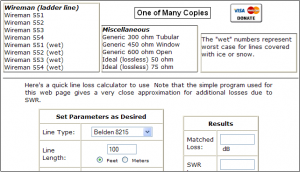That particular copy is "copyrighted" by the way. And donations are gladly accepted. But moving on ...

The disadvantage of the ARRL formula is that the results may or may not be particularly accurate. And there's no way to know without doing more rigorous calculations, starting with the basic "Transmission Line Equation" (also called the "Telegrapher's Equation") which calculates the impedance at the input end (near end) of a line given the impedance at the load (far end, termination) and the characteristics of the line itself.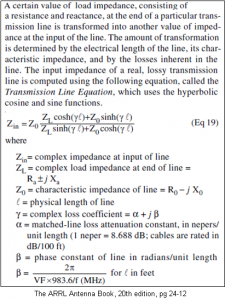Once you know the impedance at the input end there are several different ways to calculate the total dB loss. The one that has been used in all AC6LA software (except JavaScript) for the past 15 years or so was described by Charles Michaels, W7XC (SK), in the November 1997 issue of QST.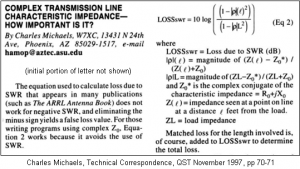Note that "LOSSswr" calculated above can be negative in some cases. That's not the same as a negative SWR which happens when the magnitude of the (normal) reflection coefficient is greater than one.

Another method was described by Steve Stearns, K6OIK, in a presentation titled "A Transmission Line Power Paradox and Its Resolution" given at Pacificon 2014. Here's page 43 from the K6OIK presentation.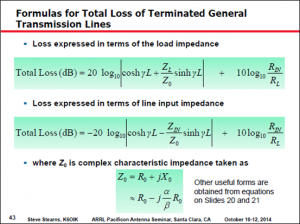The full K6OIK presentation can be downloaded here. It includes a very nice explanation of the flawed assumption in the ARRL formula and why the formula is accurate only in special cases, none of which have anything to do with the stated warning of "SWR levels less than 20:1".

Yet another method is convenient if you also want to calculate the current and voltage at either end of the line. The lower formulas below calculate the current at the load (termination) based on the current at the input; on the left using the impedance at the load, on the right using the impedance at the input.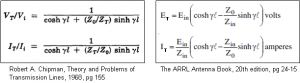Given an arbitrary current at the input end of the line, say 1 amp amplitude at 0° phase, the above formulas give the current at the load (termination). Using those values you can compute the power levels at each end of the line:

Pin = |Iin|^2 * Rin

Then the dB loss is:

Total line loss (dB) = 10 * Log10(Pin / Pload)

Still another way to compute total line loss is to use the "lossy transmission line" feature of EZNEC v. 5.0.EZNEC will calculate the impedance (and power) at the source, the input end of the line. EZNEC will also calculate the current at the antenna feedpoint, the load end of the line. Knowing the feedpoint current along with the feedpoint resistance you can calculate the power at the load. With Pin and Pload you can calculate total line loss in dB as shown previously.

So how do all these methods compare? Rather than using some arbitrary load impedance let's examine an 80m dipole that has been cut slightly long and is fed with RG-8X. In particular, at 3.5 MHz a 140 ft #12 wire at a height of 30 ft over average ground has a modeled feedpoint impedance of 54.52+j62.84 ohms. With that load impedance and using transmission line characteristics typical for RG-8X here is a plot of the calculated total line loss for line lengths from 5 to 200 ft.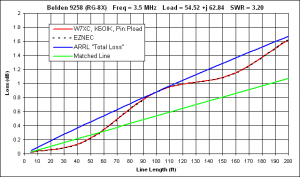The W7XC, K6OIK, and Pinload formulas all produce identical results. The EZNEC "lossy transmission line" values differ from the more precise values by an average of 0.004 dB, barely detectable on the plot. On the other hand, the ARRL "Total Loss" formula is accurate only by happenstance at certain line lengths. And note that the SWR at the load is 3.20:1, not at all extreme.

Also interesting is that for this combination of load, transmission line, and frequency, the total power loss is actually less than the "matched line" loss at lengths below about 55 ft. That's a tough concept to grasp if you've always thought of "matched line loss" as being equivalent to "minimum loss".

Now in all fairness we need to dig a little deeper. The discrepancy between the ARRL approximation and the more accurate calculations is due to the reactive component of the line's characteristic impedance; the Xo part of Zo=Ro-jXo. If Xo is arbitrarily set to zero then the ARRL formula matches the more accurate calculations exactly.

The characteristic impedance Zo of real transmissions lines at frequencies below the GHz range always has a -jXo component, but Xo decreases in magnitude (gets closer to zero) at higher frequencies and for better quality lines with less inherent loss. So suppose we scale the slightly-long dipole up to 14 MHz and feed it with RG-213. Assuming the antenna stays at a height of 30 ft the feedpoint impedance will be 84.51+j28.50 ohms. And with that load impedance at the end of 5 to 200 ft of RG-213 here's the total line loss.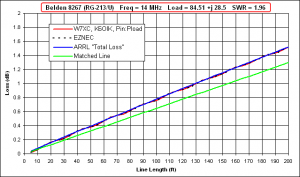Pretty close. But of course you wouldn't know that unless you did the exact calculations. And there are plenty of tools available to help.

(Continued in Part 2 below.)

Dan, AC6LA

2. Part 2 - Use of various tools to calculate total line loss

Here are several different tools that will do all the messy math for you. Each example shown is for the 80m dipole at 3.5 MHz with a feedpoint impedance of 54.52+j62.84 ohms fed with 40 ft of RG-8X.

First up is TLDetails. Select a line type and enter the frequency, load R/X, and line length.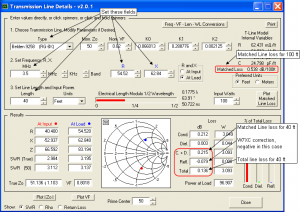Or you could use the TLW program by N6BV. The specs for RG-8X that are built-in to TLW are not quite the same as those used by TLDetails, but you can set a "User-Defined" transmission line to allow comparison with other tools. Or you could use the TLW RG-8X specs in the other tools. There is no "Gospel Truth" set of specifications for any given transmission line type.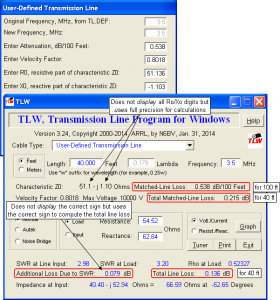Since you need to know the load impedance (the impedance at the antenna feedpoint) anyway, you can use EZNEC v. 5.0 to provide all the data required to calculate the total line loss as well. First create a model with no transmission line (source at the antenna feedpoint) and look at the "Source Data" window. Make a note of the resistance value. Then add a transmission line to the model and recalculate. Now look at the "Source Data" window again to get the power in to the transmission line. Then look at the "Currents" window to get the magnitude of the current at the antenna feedpoint, the load end of the line. Use that value along with the previously-calculated feedpoint resistance to calculate the power at the load end of the transmission line. Then use the power values to calculate the total line loss in dB.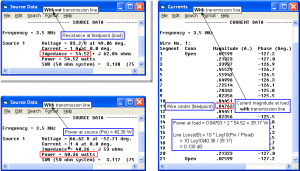Call that Method A; it's just pocket calculator math. But there's an even easier Method B. In the first model, without a transmission line, make a note of the "Slice Max Gain" as shown in the 2D Plot window. Then add the transmission line and note the new "Slice Max Gain". The difference between those two values is the power that was lost in the line. The value will be limited to two decimal places because that's the limit on gain shown by EZNEC. Don't lose any sleep over that.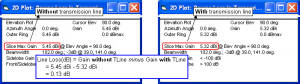If you have AutoEZ you can do everything at once, plus calculations for multiple line lengths, plus let Excel do all the math. Create the wire(s) for your model, put a 1 amp current source at V1, then define a transmission line with End 1 at V1 and End 2 at the antenna feedpoint. Set the length using a variable, "=L" in the illustration below. Then click the "Set Zo, VF, and Loss" button. Select from the list of approximately 100 built-in line types or set the wire diameter and spacing for custom parallel conductor line. When you click OK the remaining fields for the transmission line will be filled in.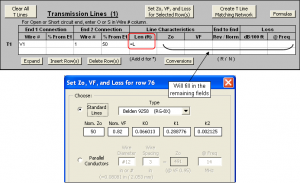On the Calculate sheet set up a series of test cases for different line lengths (variable "L") with the first value being a "virtual zero" length. You can't set the length to exactly zero but 0.001 ft is essentially the same thing. Thus the first test case is equivalent to "without transmission line" and the remaining test cases are "with transmission line" at increasing line lengths.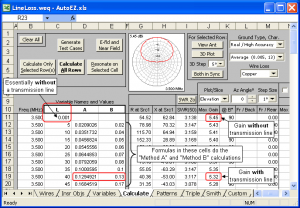The cell formulas for variables "A" and "B" do the calculations described as Method A and Method B earlier. (A sample model is available showing the Method A formula although the simple subtraction of Method B is perfectly adequate.) After you calculate all rows you can tab to the Custom sheet and plot "Variable 2" ("A") or "Variable 3" ("B") vs "Variable 1" ("L", line length).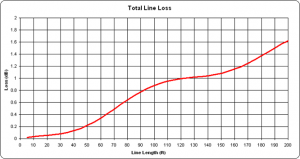If you are not a user of EZNEC or AutoEZ you can still plot the loss for multiple line lengths as long as you have Microsoft Excel (not an Excel clone). Use the small workbook that I created for this project. It includes a library of line types with the same specifications as TLDetails and AutoEZ. All you have to do is set the frequency, choose a line type, and set the load R/X values.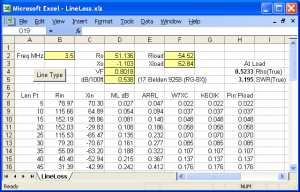The workbook includes a chart that automatically updates as you make changes and it can be interesting to see the effect of changing the load R/X. For example, here is the situation if Xload is set as negative 62.84 ohms. Notice that the precise values are now on the "other side" of the ARRL loss values.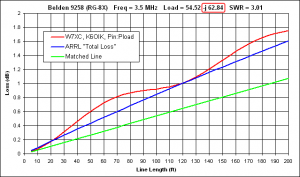If you enjoy noodling around with Excel you are more than welcome to look under the hood to see how things were done.

Resources

TLDetails: http://ac6la.com/tldetails1.html

TLW: Bundled with the ARRL Antenna Book

EZNEC: http://eznec.com/

AutoEZ: http://ac6la.com/autoez.html

Sample AutoEZ model "LineLoss.weq" (requires AutoEZ v2.0.13 or later): http://ac6la.com/adhoc/LineLoss.weq

"LineLoss.xls" Excel workbook (requires Excel, with macros enabled): http://ac6la.com/LineLoss.xls

Even more transmission line math: http://ac6la.com/tlmath.html

Dan, AC6LA

3. ### KL7AJXML SubscriberQRZ Page

G3TXQ demonstrated how the additional loss concept requires that "a few" wavelengths of line are necessary for the accepted formulas to hold. The famous "Q" section when terminated in a very high impedance (such as the end of a Zepp antenna) actually has less loss than a matched line.Great stuff.....amazing how elaborate something like a pair of wires can be!

4. I love it when Dan provides just a "few" notes on a subject.

5. You should have seen the unabridged version!

Dan, AC6LA

6. ### KL7AJXML SubscriberQRZ Page

You wouldn't believe the flak I got when I wrote "SWR METERS MAKE YOU STUPID" because it was 14 pages long. You'd think I made them read War and Peace in Cyrillic!

7. Eric, don't you realize that it's unfair to discriminate against the technically challenged by forcing them to read your postings?8. i haven't looked at the presentation by K6OIK, but in reviewing the calculated results in graph form, i'm inclined to believe it is more accurate.

loss must increase as line length increases because resistive losses increase. but, since we're dealing with the concept of reflections, there are specific lengths that must provide less loss due to the additive nature of proper phasing.

just this crackpots opinion.

9. obtw i think you might want to recheck your plot for the pin/pload. the sinusoid appears inverted (compared to the earlier graph)